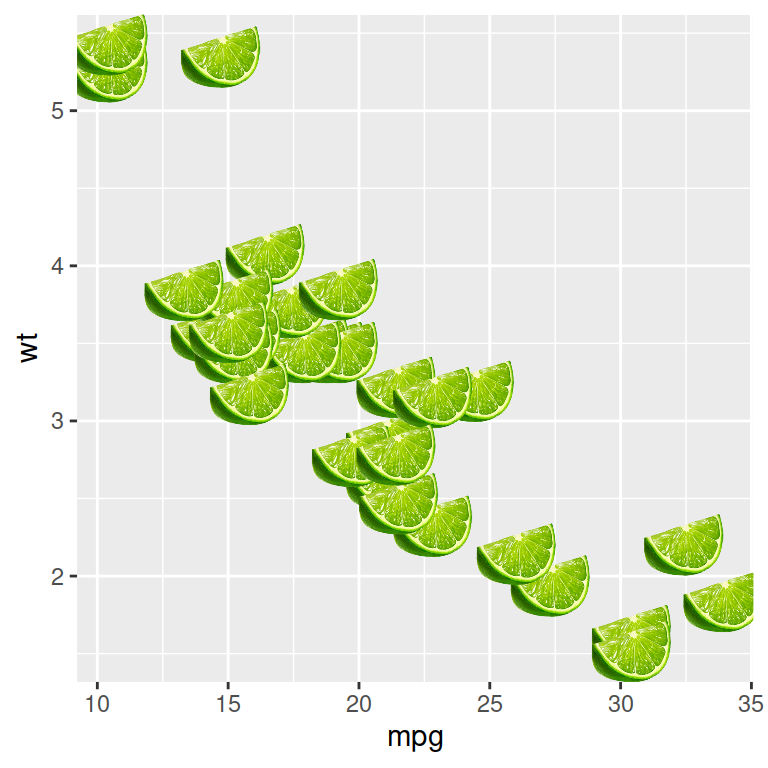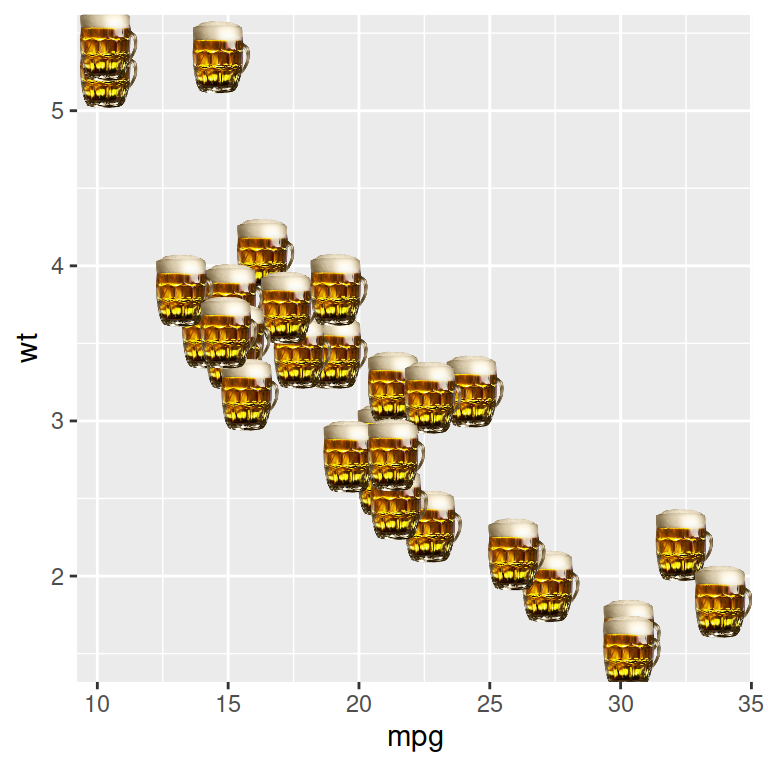# geom_lime and geom_pint

coolbutuseless

## `geom_lime`

The `geom_lime` is a ggplot geom that draws limes instead of points (when you misspell `geom_line`). Note that it is possible to modify the size of the limes with `size`.

``````# install.package('remotes')
# remotes::install_github("coolbutuseless/geomlime")
library(geomlime)
# install.packages("ggplot2")
library(ggplot2)

ggplot(mtcars, aes(mpg, wt)) +
geom_lime(size = 6)``````## `geom_pint`The `geomlime` package comes with another funny geom called `geom_pint`, which draws pints instead of points (when you misspell `geom_point`).

``````# install.package('remotes')
# remotes::install_github("coolbutuseless/geomlime")
library(geomlime)
# install.packages("ggplot2")
library(ggplot2)

ggplot(mtcars, aes(mpg, wt)) +
geom_pint(size = 6)``````